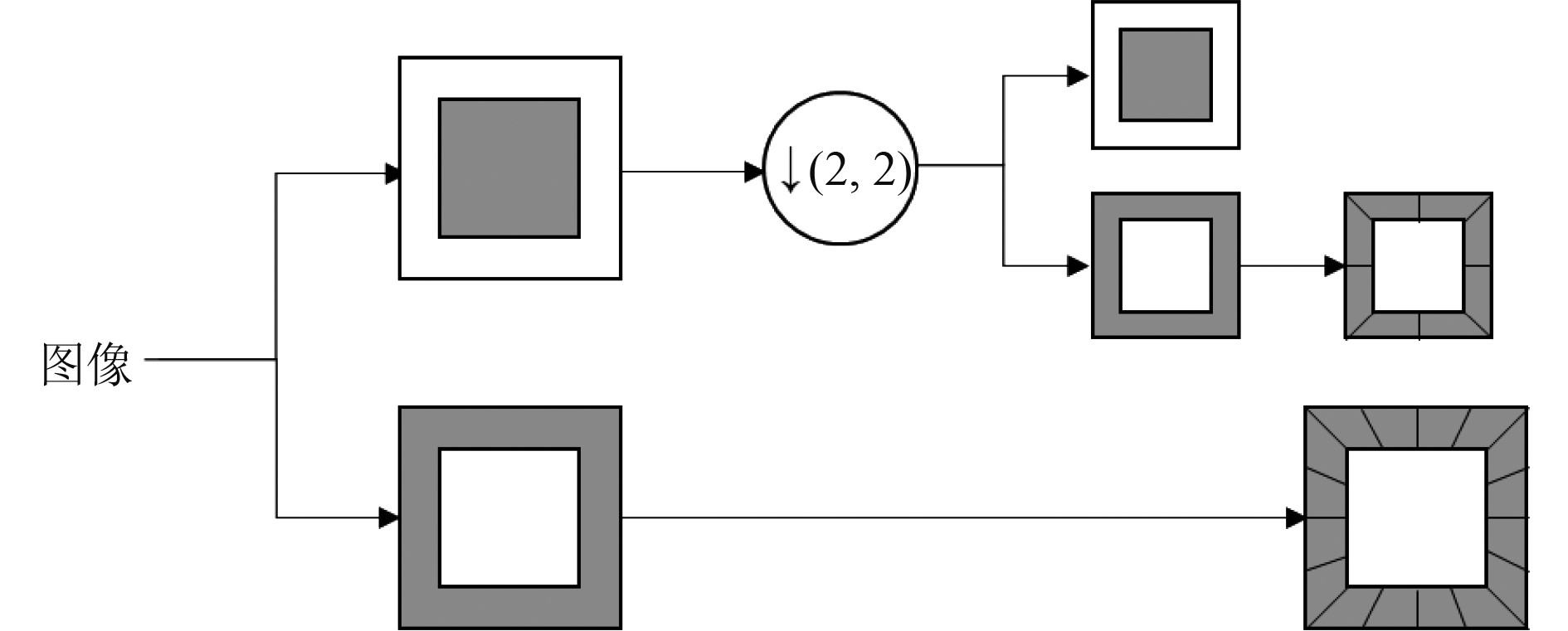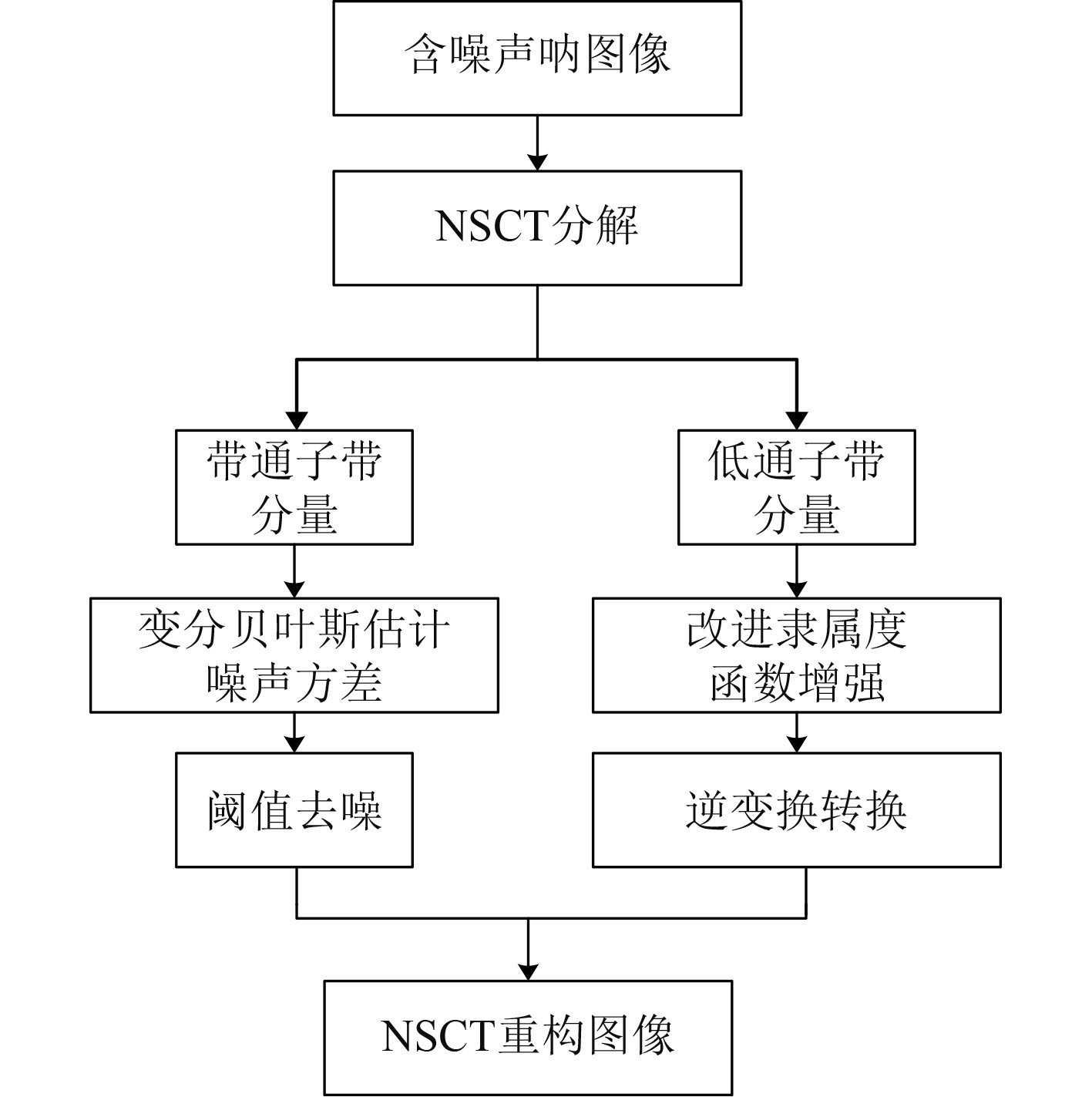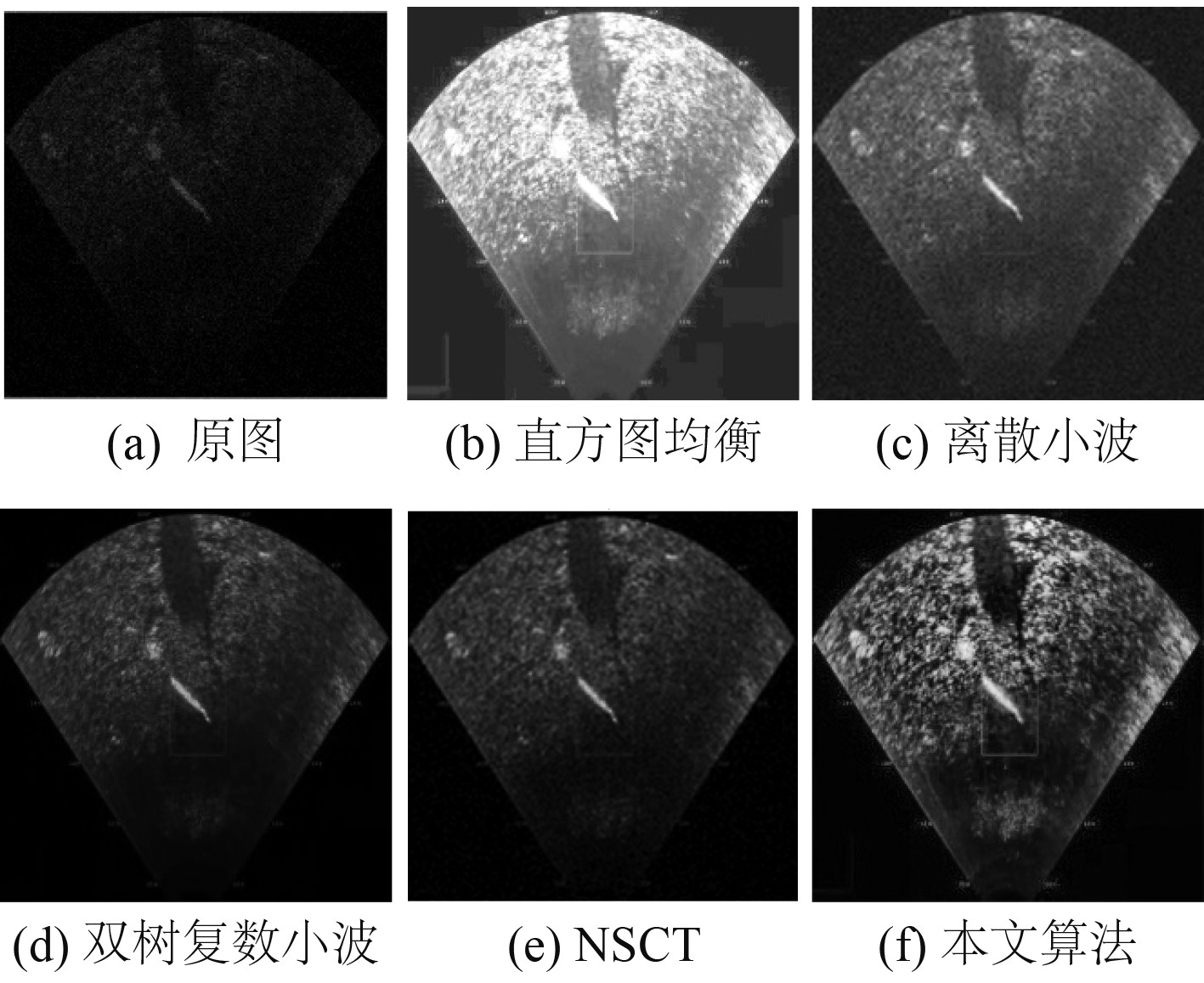﻿ 联合NSCT模糊域的声呐图像目标增强方法研究
 舰船科学技术2022, Vol. 44Issue (7): 138-141    DOI: 10.3404/j.issn.1672-7649.2022.07.027PDF

, 曾庆军1, 胡健阳2
1. 江苏科技大学 电子信息学院，江苏 镇江 212000;
2. 江苏科技大学 计算机学院，江苏 镇江 212000

Research on sonar image target enhancement method combined with NSCT fuzzy domain
, ZENG Qin-jun1, HU Jian-yang2
1. School of Electronic Information, Jiangsu University of Science and Technology, Zhenjiang 212000, China;
2. School of Computer Science, Jiangsu University of Science and Technology, Zhenjiang 212000, China
Abstract: A non - subsampled contourlet transform (NSCT) combined with adaptive threshold is proposed for noise suppression and target enhancement. First, NSCT can be used to decompose noise-containing sonar images into different frequency and directional response subbands. Then, we use the adaptive threshold operator to de-noise the subband of the NSCT coefficient. Combined with the feature of field information and spatial information to construct the fuzzy, to build up the fuzzy membership degree after the contraction function of noise reduction of NSCT secondary noise component implementation, and finally to rebuild the sonar image data of the noise reduction after using sample data to prove that the proposed method can effectively remove noise sonar image, and is superior to the threshold denoising method based on wavelet transform.
Key words: sonar image     NSCT     fuzzy domain     translational invariance     V-Bayes
0 引　言

1 相关介绍 1.1 非下采样轮廓波NSCT

Contourtlet变换可以以最佳方式稀疏地表示图像，并且能有效捕获和刻画图像中弯曲和定向的几何结构，其结构中LP和DFB都进行了下采样操作，因此Contourtlet系数的冗余大大降低，以至于Contourlet 变换缺少平移不变性，使增强后的图像在不连续的点边缘产生伪“Gibbs”现象。为了解决上述问题，NSCT舍弃了Contourtlet变换中的下采样操作，将非下采样金字塔（NSP）和非下采样方向滤波器组（NSDFB）组合，在采样的比例上与原始图像比例相同。

NSP可确保NSCT的多尺度特性，NSP分解阶段生成2个子带：低频子带和高频子带，利用NSDFB对高频子带进行方向分析，从各个方向展示图像的轮廓细节和边缘特征。NSP对图像进行n级尺度分解，可得到n+1个子带图像。图1为2层NSCT变换的原理图。图 1 NSCT分解原理图 Fig. 1 NSCT decomposition schematic
1.2 双变量模型

NSCT分解相邻尺度之间的各级系数有很强的相关性，即NSCT变换域中的系数具有与小波系数相似的分布特性。因此，小波域中的双变量模型的处理思想可用于将其扩展到NSCT变换域中。

 $y = w + n,$ (1)

 $\hat w(y) = {\rm{argmax}}[{\rm{log}}({p_n}(y{\text{ - }}w)) + {\rm{log}}({p_n}(w))]。$ (2)

 ${p_n}(n) = \frac{1}{{2{\text{π}} \sigma _n^2}}{\rm{exp}}\left( - \frac{{n_1^2 + n_2^2}}{{2\sigma _n^2}}\right) 。$ (3)

Sendur等提出双变量模型对小波上下级系数进行描述，NSCT内系数概率分布用联合概率密度函数 ${p}_{w}$ 表示：

 ${p_w}(w) = \frac{3}{{2{\text{π}} {\sigma ^2}}}{\rm{exp}}( - \frac{{\sqrt 3 }}{\sigma }\sqrt {w_1^2 + w_2^2} ),$ (4)

 ${\hat w_1} = \frac{{\left(\sqrt {y_1^2 + y_2^2} - \dfrac{{\sqrt 3 \sigma _n^2}}{\sigma }\right)}}{{\sqrt {y_1^2 + y_2^2} }} + {y_1} 。$ (5)

1.3 变分贝叶斯阈值估计

MAP估计方法不需要显示求出后的后验密度函数，只要得出最大概率密度的点值，属于点估计范畴，对模型参数没有给出一个很好的估计准则，而变分贝叶斯方法是对整个后验密度的求解，因此可以利用更多的信息.为了解决传统贝叶斯估计问题，变分贝叶斯方法提供了有效的解决方案。

 $p\left( {\nabla \left. w \right|\nabla y} \right) = \frac{{p\left( {\nabla \left. y \right|\nabla w} \right)p\left( {\nabla w} \right)}}{{p\left( {\nabla y} \right)}}。$ (6)

 $\begin{split} &KL\left\{q(\nabla w,{\sigma }^{2})\Vert p(\nabla w|\nabla y)\right\}=\\ &\qquad \int q(\nabla w,{\sigma }^{2})\mathrm{ln}\dfrac{q(\nabla w,{\sigma }^{2})}{p(\nabla w|\nabla y)}{\rm{d}}\nabla w+\mathrm{ln}p(\nabla y)\geqslant 0，\end{split}$ (7)

$p(\nabla y)$ 在整个估计过程中始终是常数，因此可以定义成本函数 ${C}_{KL}$ 以获得近似分布的最佳值：

 $\begin{split} {C}_{KL}=&KL\{q(\nabla w,{\sigma }^{2})\Vert p(\nabla w|\nabla y)\}-\mathrm{ln}p(\nabla y)=\\ & \int q(\nabla w,{\sigma }^{2})\mathrm{ln}\dfrac{q(\nabla w,{\sigma }^{2})}{p(\nabla w|\nabla y)}{\rm{d}}\nabla w+\\ & \int q(-{\sigma }^{2})\mathrm{ln}\dfrac{q(-{\sigma }^{2})}{p(-{\sigma }^{2})}{\rm{d}}(-{\sigma }^{2})。\end{split}$ (8)

2 模糊对比度增强

NSCT分解后，其低频子带具有 $L$ 个灰度级的 $M\times N$ 图像，可以看作为一个模糊集，其每一个元素对应的模糊特征集合，记为模糊矩阵：

 $X = \mathop \bigcup \limits_{m = 1}^M \mathop \bigcup \limits_{n = 1}^N \frac{{{u_{mn}}}}{{{x_{mn}}}} ,$ (9)

 ${u_{mn}} = \frac{1}{2}\left[{\rm{sin}}\left(\frac{{\text{π} ({x_{mn}} - {x_{{\rm{min}}}})}}{{2({x_{{\rm{max}}}} - {x_{{\rm{min}}}})}}\right) - {\rm{cos}}\left(\frac{{\text{π} ({x_{mn}} - {x_{{\rm{min}}}})}}{{2({x_{{\rm{max}}}} - {x_{{\rm{min}}}})}}\right) + 1\right] 。$ (10)

 $\begin{split} u_{mn}^{'} =& {T^r}\left( {{u_{mn}}} \right) = T\left( {{T^{r - 1}}\left( {{u_{mn}}} \right)} \right) = \\ &\frac{{{\rm{sin}}(\text{π} ({u_{mn}}{{ - }}0.5)) + 1}}{2}。\end{split}$ (11)

 ${x_{mn}} = {x_{{\rm{min}}}} + ({x_{{\rm{max}}}} - {x_{{\rm{min}}}})\frac{{{\rm{arcsin}}{{[1 - {{\left( {2{u_{mn}} - 1} \right)}^2}]}^{\sqrt r }}}}{{\text{π}}} 。$ (12)
2.1 具体步骤

NSCT具有多方向选择性和平移不变性的优点，而双变量模型可以充分利用图像小波系数的尺度间相关性。图 2 图像增强方法流程图 Fig. 2 The image enhancement method flow chart
3 实验对比分析表 1 五种算法的客观指标 Tab.1 Objective index of five algorithms图 3 前视声呐图像5种增强方法的结果比较 Fig. 3 Comparison of results of five enhancement methods for forward-looking sonar images

4 结　语

  陈鹏, 张建伟. 结合核函数与非线性偏微分方程的图像去噪方法[J]. Computer Science, 2018, 45(11): 278-282. DOI:10.11896/j.issn.1002-137X.2018.11.044  WANG Y, WANG H, YIN C, et al. Biologically inspired image enhancement based on Retinex[J]. Neurocomputing, 2016, 177(177): 373-384.  杨蕴, 李玉, 王玉. 一种数学形态学的量子图像去噪方法[J]. 遥感信息, 2018, 33(2): 17-25. DOI:10.3969/j.issn.1000-3177.2018.02.003  李利, 杨恢先, 何雅丽, 等. 结合FT与NSCT的自适应阈值去噪算法[J]. 计算机工程, 2012, 38(17): 238-241. DOI:10.3778/j.issn.1002-8331.2012.17.048  姜三平, 郝晓剑. 应用小波系数GSM统计模型的混合傅里叶-小波图像降噪[J]. 中国图象图形学报, 2009, 14(3): 448-451.  MA Guangsheng, ZHAO Jiman. Quaternion ridgelet transform and curvelet transform. [J].Adoances in Applied Clifford Algebras, 2018, 28(4): 1-21.  SHAHDOOSTI H R, HAZAVEI S M. Image denoising in dual contourlet domain using hidden Markov tree models[J]. Digital Signal Processing, 2017, 17-29.  STARCK J L , CANDES E J , DONOHO D L . The curvelet transform for image denoising[J]. IEEE Transaction on image processing a publication of the IEEE signal processing society, 2002, 11(6): 670-684.  DA, C, ARTHUR, et al. The nonsubsampled contourlet transform: theory, design, and applications.[J]. IEEE Transactions on Image Processing, 2006, 15(10): 3089-3101. DOI:10.1109/TIP.2006.877507  吴炜, 杨晓敏, 陈默, 等. 基于改进的非下采样Contourlet变换的超分辨率复原算法[J]. 光学学报, 2009, 29(6): 1493-1501.  HUANG Yun-hu, CHEN De-wang. Image fuzzy enhancement algorithm based on contourlet transform domain[J]. Multimedia Tools and Applications, 2019.  杨建雷. 基于非采样Contourlet变换和模糊逻辑的图像增强算法研究[D]. 保定:河北大学, 2011.  FRAYSSE A, RODET T . A measure-theoretic variational Bayesian algorithm for large dimensional problems[J]. SIAM Journal on Imaging Sciences, 2013, 7(4).  霍冠英, 李庆武, 王敏, 等. Curvelet域贝叶斯估计侧扫声呐图像降斑方法[J]. 仪器仪表学报, 2011, 32(1): 170-177.  李庆武, 马国翠, 霍冠英, 等. 基于NSCT域边缘检测的侧扫声呐图像分割新方法[J]. 仪器仪表学报, 2013(8): 1795-1801. DOI:10.3969/j.issn.0254-3087.2013.08.016  LYU Zhiyu, ZHANG Chengkun, HAN Min. A nonsubsampled countourlet transform based CNN for real image denoising[J]. Signal Processing:Image Communication, 2020, 82.  LU Huimin, YAMAWAKI A, Serikawa S. Curvelet approach for deep-sea sonar image denoising, contrast enhancement and fusion[J]. Journal of International Council on Electrical Engineering, 2013, 3(3).